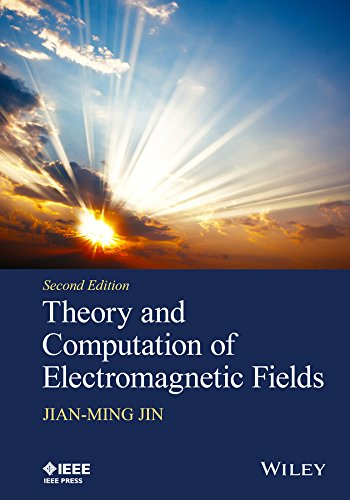Sale!

# Theory and Computation of Electromagnetic Fields (Wiley IEEE) 2nd Edition Ebook PDF Version

N/A

### Theory and Computation of Electromagnetic Fields (Wiley IEEE) 2nd EditionReviews the fundamental concepts behind the theory and computation of electromagnetic fields

The book is divided in two parts. The first part covers both fundamental theories (such as vector analysis, Maxwell’s equations, boundary condition, and transmission line theory) and advanced topics (such as wave transformation, addition theorems, and fields in layered media) in order to benefit students at all levels. The second part of the book covers the major computational methods for numerical analysis of electromagnetic fields for engineering applications. These methods include the three fundamental approaches for numerical analysis of electromagnetic fields: the finite difference method (the finite difference time-domain method in particular), the finite element method, and the integral equation-based moment method. The second part also examines fast algorithms for solving integral equations and hybrid techniques that combine different numerical methods to seek more efficient solutions of complicated electromagnetic problems.

Theory and Computation of Electromagnetic Fields, Second Edition:

• Provides the foundation necessary for graduate students to learn and understand more advanced topics
• Discusses electromagnetic analysis in rectangular, cylindrical and spherical coordinates
• Covers computational electromagnetics in both frequency and time domains
• Includes new and updated homework problems and examples

Theory and Computation of Electromagnetic Fields, Second Edition is written for advanced undergraduate and graduate level electrical engineering students. This book can also be used as a reference for professional engineers interested in learning about analysis and computation skills.
ISBN 9781119108047

\$174.00 \$23.99Add to Wishlist
ISBN-13 9781119108047 N/A N/A N/A

### Theory and Computation of Electromagnetic Fields (Wiley IEEE) 2nd EditionReviews the fundamental concepts behind the theory and computation of electromagnetic fields

The book is divided in two parts. The first part covers both fundamental theories (such as vector analysis, Maxwell’s equations, boundary condition, and transmission line theory) and advanced topics (such as wave transformation, addition theorems, and fields in layered media) in order to benefit students at all levels. The second part of the book covers the major computational methods for numerical analysis of electromagnetic fields for engineering applications. These methods include the three fundamental approaches for numerical analysis of electromagnetic fields: the finite difference method (the finite difference time-domain method in particular), the finite element method, and the integral equation-based moment method. The second part also examines fast algorithms for solving integral equations and hybrid techniques that combine different numerical methods to seek more efficient solutions of complicated electromagnetic problems.

Theory and Computation of Electromagnetic Fields, Second Edition:

• Provides the foundation necessary for graduate students to learn and understand more advanced topics
• Discusses electromagnetic analysis in rectangular, cylindrical and spherical coordinates
• Covers computational electromagnetics in both frequency and time domains
• Includes new and updated homework problems and examples

Theory and Computation of Electromagnetic Fields, Second Edition is written for advanced undergraduate and graduate level electrical engineering students. This book can also be used as a reference for professional engineers interested in learning about analysis and computation skills.
ISBN 9781119108047

## Reviews

There are no reviews yet.